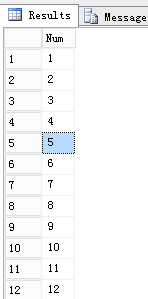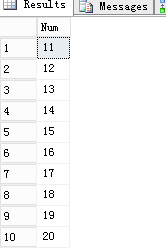�����ڵ�λ�ã���ҳ >> ���ݿ� >> SQLServer >> ����

SQL Server ʵ�����ָ�����ʵ������--Javaѧϰ��

ʱ�䣺2016-11-10 8:17:19

����������ʾ�����ָ�������һ���������������У�ͨ������ʵ�ֶ��ֲ�ͬ�Ĳ�ѯ���񡣴������ࣺ�㹻���������ֱ��ͱ�������ǰ�߿��Գ�Ϊ��̬�ģ����߿��Գ�Ϊ��̬�Ұ����������������ֱ� �������ֱ�ͨ������һ��������������¼��...

���ָ�������һ���������������У�ͨ������ʵ�ֶ��ֲ�ͬ�Ĳ�ѯ���񡣴������ࣺ�㹻���������ֱ��ͱ�������ǰ�߿��Գ�Ϊ��̬�ģ����߿��Գ�Ϊ��̬�Ұ���������

�������ֱ�

�������ֱ�ͨ������һ��������������¼����㹻����ص�T-SQL�������£�

 1 2 3 4 5 6 7 8 9 10 11 12 13 14 15 16 17 18 19 20 `IF OBJECT_ID(N``'dbo.Nums'``, ``'U'``) ``IS` `NOT` `NULL` `BEGIN` `  ``DROP` `TABLE` `dbo.Nums;` `END` `GO` ` `  `CREATE` `TABLE` `dbo.Nums ` `(` `  ``Num ``INT` `NOT` `NULL``,` `  ``CONSTRAINT` `PK_U_CL_Nums_Num ``PRIMARY` `KEY` `CLUSTERED` `  ``(` `    ``Num ``ASC` `  ``) ` `);` `GO` ` `  `INSERT` `INTO` `dbo.Nums (Num)` `SELECT` `ROW_NUMBER() OVER (``ORDER` `BY` `(``SELECT` `NULL``)) ``AS` `RowNum` `FROM` `master.dbo.spt_values;` `GO`

ע�⣺�������������ֱ��ķ����ܶ࣬Ϊ����ʾ����ʹ����һ�֡�

���Ե�T-SQL�������£�

 1 2 3 `1 ``SELECT` `Num` `2 ``FROM` `dbo.Nums;` `3 GO`

ִ�к�Ĳ�ѯ������£�������

������ʵ��ʹ�ý������Ӻ�CTE��SQL Server 2005�����ϰ汾��T-SQL�������£�

 1 2 3 4 5 6 7 8 9 10 11 12 13 14 15 16 17 18 19 20 21 22 23 24 25 26 27 28 29 30 31 32 33 34 `IF OBJECT_ID(N``'dbo.ufn_GetNums'``, N``'IF'``) ``IS` `NOT` `NULL` `BEGIN` `  ``DROP` `TABLE` `dbo.ufn_GetNums;` `END` `GO` ` `  `--==================================` `-- ����: ��ȡָ����Χ����������` `-- ˵��: �������㼶��CTE�õ��������У���L������0��ʼ�������õ����е�����Ϊ2^2^L��` `--    ���磺��5���ͻ�õ�4 294 967 596�С�5����CTE�ṩ�˳���40�ڵ��С�` `-- ����: XXX` `-- ����: yyyy-MM-dd` `-- �޸�: yyyy-MM-dd XXX �޸���������` `--==================================` `CREATE` `FUNCTION` `dbo.ufn_GetNums` `(` `  ``@bintLow ``BIGINT``,` `  ``@bintHigh ``BIGINT` `) ``RETURNS` `TABLE` `AS` `RETURN` `  ``WITH` `    ``L0 ``AS` `(``SELECT` `c ``FROM` `(``VALUES``(1), (1)) ``AS` `LO(c)),` `    ``L1 ``AS` `(``SELECT` `1 ``AS` `c ``FROM` `L0 ``AS` `T ``CROSS` `JOIN` `L0 ``AS` `T2),` `    ``L2 ``AS` `(``SELECT` `1 ``AS` `c ``FROM` `L1 ``AS` `T ``CROSS` `JOIN` `L1 ``AS` `T2),` `    ``L3 ``AS` `(``SELECT` `1 ``AS` `c ``FROM` `L2 ``AS` `T ``CROSS` `JOIN` `L2 ``AS` `T2),` `    ``L4 ``AS` `(``SELECT` `1 ``AS` `c ``FROM` `L3 ``AS` `T ``CROSS` `JOIN` `L3 ``AS` `T2),` `    ``L5 ``AS` `(``SELECT` `1 ``AS` `c ``FROM` `L4 ``AS` `T ``CROSS` `JOIN` `L4 ``AS` `T2),` `    ``Nums ``AS` `(``SELECT` `ROW_NUMBER() OVER (``ORDER` `BY` `(``SELECT` `NULL``)) ``AS` `RowNum ``FROM` `L5)` ` `  `  ``SELECT` `TOP` `(@bintHigh - @bintLow + 1) @bintLow + RowNum - 1 ``AS` `Num` `  ``FROM` `Nums` `  ``ORDER` `BY` `RowNum ``ASC``;` `GO`

SQL Server 2012�������йط�ҳ�������ԣ���ص�T-SQL��������:

 1 2 3 4 5 6 7 8 9 10 11 12 13 14 15 16 17 18 19 20 21 22 23 24 25 26 27 28 29 30 31 32 33 34 35 `IF OBJECT_ID(N``'dbo.ufn_GetNums2'``, N``'IF'``) ``IS` `NOT` `NULL` `BEGIN` `  ``DROP` `TABLE` `dbo.ufn_GetNums2;` `END` `GO` ` `  `--==================================` `-- ����: ��ȡָ����Χ����������` `-- ˵��: �������㼶��CTE�õ��������У���L������0��ʼ�������õ����е�����Ϊ2^2^L��` `--    ���磺��5���ͻ�õ�4 294 967 596�С�5����CTE�ṩ�˳���40�ڵ��С� ` `-- ����: XXX` `-- ����: yyyy-MM-dd` `-- �޸�: yyyy-MM-dd XXX �޸���������` `--==================================` `CREATE` `FUNCTION` `dbo.ufn_GetNums2` `(` `  ``@bintLow ``BIGINT``,` `  ``@bintHigh ``BIGINT` `) ``RETURNS` `TABLE` `AS` `RETURN` `  ``WITH` `    ``L0 ``AS` `(``SELECT` `c ``FROM` `(``VALUES``(1), (1)) ``AS` `LO(c)),` `    ``L1 ``AS` `(``SELECT` `1 ``AS` `c ``FROM` `L0 ``AS` `T ``CROSS` `JOIN` `L0 ``AS` `T2),` `    ``L2 ``AS` `(``SELECT` `1 ``AS` `c ``FROM` `L1 ``AS` `T ``CROSS` `JOIN` `L1 ``AS` `T2),` `    ``L3 ``AS` `(``SELECT` `1 ``AS` `c ``FROM` `L2 ``AS` `T ``CROSS` `JOIN` `L2 ``AS` `T2),` `    ``L4 ``AS` `(``SELECT` `1 ``AS` `c ``FROM` `L3 ``AS` `T ``CROSS` `JOIN` `L3 ``AS` `T2),` `    ``L5 ``AS` `(``SELECT` `1 ``AS` `c ``FROM` `L4 ``AS` `T ``CROSS` `JOIN` `L4 ``AS` `T2),` `    ``Nums ``AS` `(``SELECT` `ROW_NUMBER() OVER (``ORDER` `BY` `(``SELECT` `NULL``)) ``AS` `RowNum ``FROM` `L5)` ` `  `  ``SELECT` `@bintLow + RowNum - 1 ``AS` `Num` `  ``FROM` `Nums` `  ``ORDER` `BY` `RowNum ``ASC` `  ``OFFSET 0 ``ROWS` `FETCH` `FIRST` `@bintHigh - @bintLow + 1 ``ROWS` `ONLY``;` `GO`

�Ժ���ufn_GetNumsΪ������ʾ��ص�Ч������ȡָ����Χ���������е�T-SQL�������£�

 1 2 3 `SELECT` `Num` `FROM` `dbo.ufn_GetNums(11, 20);` `GO`

ִ�к�Ĳ�ѯ������£���ע΢�źţ�javalearns   ��ʱ���ѧJava

��ɨһɨ��ʱ���ѧJava

���ߣ����ꡡ��Դ������
���Ǵ�����֪������վ��?
• ���ѽ��ܵ�
• �ٶ�������
• ��������������
• ��ַ����˽�����
• ̫æ�˲��ǵ����������� 0 ��������������ҵ�����• ������
• ���ݣ�
• û��
����̶�
• û��
•